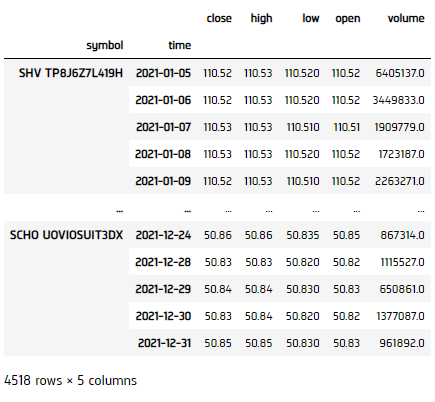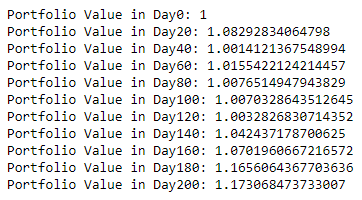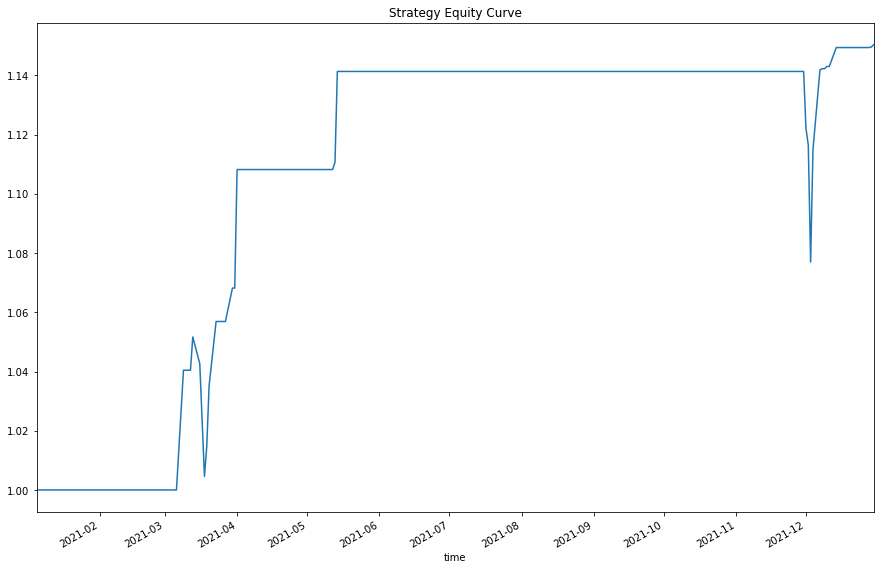# Applying Research

## Mean Reversion

### Introduction

This page explains how to you can use the Research Environment to develop and test a Mean Reversion hypothesis, then put the hypothesis in production.

### Create Hypothesis

Imagine that we've developed the following hypothesis: stocks that are below 1 standard deviation of their 30-day-mean are due to revert and increase in value, statistically around 85% chance if we assume the return series is stationary and the price series is a Random Process. We've developed the following code in research to pick out such stocks from a preselected basket of stocks.

### Import Libraries

Load the required assembly files and data types.

We'll need to import libraries to help with data processing. Import numpy and scipy libraries by the following:

#load "../Initialize.csx"

using QuantConnect;
using QuantConnect.Data;
using QuantConnect.Data.Market;
using QuantConnect.Algorithm;
using QuantConnect.Research;
using System;
using MathNet.Numerics.Distributions;
import numpy as np
from scipy.stats import norm, zscore

### Get Historical Data

To begin, we retrieve historical data for researching.

1. Instantiate a QuantBook.
2. var qb = new QuantBook();
qb = QuantBook()
3. Select the desired tickers for research.
4. var assets = new List<string>() {"SHY", "TLT", "SHV", "TLH", "EDV", "BIL",
"SPTL", "TBT", "TMF", "TMV", "TBF", "VGSH", "VGIT",
"VGLT", "SCHO", "SCHR", "SPTS", "GOVT"};
assets = ["SHY", "TLT", "SHV", "TLH", "EDV", "BIL",
"SPTL", "TBT", "TMF", "TMV", "TBF", "VGSH", "VGIT",
"VGLT", "SCHO", "SCHR", "SPTS", "GOVT"]
5. Call the AddEquity method with the tickers, and their corresponding resolution.
6. foreach(var ticker in assets){
}
for i in range(len(assets)):
qb.AddEquity(assets[i],Resolution.Minute)

If you do not pass a resolution argument, Resolution.Minute is used by default.

7. Call the History method with qb.Securities.Keys for all tickers, time argument(s), and resolution to request historical data for the symbol.
8. var history = qb.History(qb.Securities.Keys, new DateTime(2021, 1, 1), new DateTime(2021, 12, 31), Resolution.Daily);
history = qb.History(qb.Securities.Keys, datetime(2021, 1, 1), datetime(2021, 12, 31), Resolution.Daily)### Prepare Data

We'll have to process our data to get an extent of the signal on how much the stock is deviated from its norm for each ticker.

1. Extract close prices for each Symbol from Slice data.
2. Select the close column and then call the unstack method.
3. var closes = new Dictionary<Symbol, List<Decimal>>();
foreach(var slice in history){
foreach(var symbol in slice.Keys){
if(!closes.ContainsKey(symbol)){
}
}
}
df = history['close'].unstack(level=0)
4. Get the 30-day rolling mean, standard deviation series, z-score and filtration for each Symbol.
5. Calculate the truth value of the most recent price being less than 1 standard deviation away from the mean price.
6. var rollingMean = new Dictionary<Symbol, List<double>>();
var rollingStd = new Dictionary<Symbol, List<double>>();
var filter = new Dictionary<Symbol, List<bool>>();
var zScore = new Dictionary<Symbol, List<double>>();
foreach(var kvp in closes)
{
var symbol = kvp.Key;
if(!rollingMean.ContainsKey(symbol)){
}
for (int i=30; i < closes.Values.ElementAt(0).Count; i++)
{
var slice = kvp.Value.Skip(i).Take(30);
}
}
classifier = df.le(df.rolling(30).mean() - df.rolling(30).std())
7. Calculate the expected return and its probability, then calculate the weight.
8. Get the z-score for the True values, then compute the expected return and probability (used for Insight magnitude and confidence).
9. var magnitude = new Dictionary<Symbol, List<double>>();
var confidence = new Dictionary<Symbol, List<double>>();
var weights = new Dictionary<Symbol, List<double>>();
foreach(var kvp in rollingMean)
{
var symbol = kvp.Key;
if(!magnitude.ContainsKey(symbol)){
}
for (int i=1; i < rollingMean.Values.ElementAt(0).Count; i++)
{
// Filter if trade or not
var trade = filter[symbol][i] ? 1d : 0d;
}
}
z_score = df.apply(zscore)[classifier]
magnitude = -z_score * df.rolling(30).std() / df.shift(1)
confidence = (-z_score).apply(norm.cdf)
10. Convert the weights into 2-d array.
11. Call fillna to fill NaNs with 0.
12. double[,] weight = new double[weights.Values.ElementAt(0).Count, weights.Count];
int j = 0;
foreach(var symbol in weights.Keys){
for(int i=0; i < weights[symbol].Count; i++){
weight[i, j] = weights[symbol][i];
}
j++;
}
magnitude.fillna(0, inplace=True)
confidence.fillna(0, inplace=True)
13. Get our trading weight, we'd take a long only portfolio and normalized to total weight = 1.
14. public double[,] Normalize(double[,] array)
{
for(int i=0; i < array.GetLength(0); i++)
{
var sum = 0.0;
for (int j=0; j < array.GetLength(1); j++)
{
sum += array[i, j];
}
if (sum == 0.0) continue;
for (int j=0; j < array.GetLength(1); j++)
{
array[i, j] = array[i, j] / sum;
}
}

return array;
}
weight = Normalize(weight);
weight = confidence - 1 / (magnitude + 1)
weight = weight[weight > 0].fillna(0)
sum_ = np.sum(weight, axis=1)
for i in range(weight.shape):
if sum_[i] > 0:
weight.iloc[i] = weight.iloc[i] / sum_[i]
else:
weight.iloc[i] = 0
weight = weight.iloc[:-1]

### Test Hypothesis

We would test the performance of this strategy. To do so, we would make use of the calculated weight for portfolio optimization.

1. Convert close price to 2-d array.
2. double[,] close = new double[closes.Values.ElementAt(0).Count, closes.Count];
int j = 0;
foreach(var symbol in closes.Keys){
for(int i=0; i < closes[symbol].Count; i++){
close[i, j] = decimal.ToDouble(closes[symbol][i]);
}
j++;
}
3. Get the total daily return series.
4. var totalValue = new List<double>{1.0};
var dailySum = 0.0;
for(int i=0; i < weight.GetLength(0) - 1; i++)
{
dailySum = 0.0;
for (int j=0; j < weight.GetLength(1); j++)
{
if (close[i, j] != 0 && double.IsFinite(close[i+1, j]) && double.IsFinite(close[i, j]) && double.IsFinite(weight[i, j]))
{
dailySum += weight[i, j] * (close[i+1, j] - close[i, j]) / close[i, j];
}
}
}
ret = pd.Series(index=range(df.shape - 1))
for i in range(df.shape - 1):
ret[i] = weight.iloc[i] @ df.pct_change().iloc[i + 1].T
5. Call cumprod to get the cumulative return.
6. total_ret = (ret + 1).cumprod()
7. Set index for visualization.
8. total_ret.index = weight.index
9. Display the result.
10. for(int i=0; i < totalValue.Count; i=i+5)
{
Console.WriteLine("Portfolio Value in Day{0}: {1}", i, totalValue[i]);
}
total_ret.plot(title='Strategy Equity Curve', figsize=(15, 10))
plt.show()### Set Up Algorithm

Once we are confident in our hypothesis, we can export this code into backtesting. One way to accomodate this model into research is to create a scheduled event which uses our model to pick stocks and goes long.

private List<string> _asset = new List<string>{"SHY", "TLT", "IEI", "SHV", "TLH", "EDV", "BIL",
"SPTL", "TBT", "TMF", "TMV", "TBF", "VGSH", "VGIT",
"VGLT", "SCHO", "SCHR", "SPTS", "GOVT"};

public override void Initialize()
{
// 1. Required: Five years of backtest history
SetStartDate(2014, 1, 1);

// 2. Required: Alpha Streams Models:
SetBrokerageModel(BrokerageName.AlphaStreams);

// 3. Required: Significant AUM Capacity
SetCash(1000000);

// 4. Required: Benchmark to SPY
SetBenchmark("SPY");

SetPortfolioConstruction(new InsightWeightingPortfolioConstructionModel());
SetExecution(new ImmediateExecutionModel());

foreach(var ticker in _asset)
{
}

// Set Scheduled Event Method For Our Model
Schedule.On(DateRules.EveryDay(),
TimeRules.BeforeMarketClose("SHY", 5),
EveryDayBeforeMarketClose);
}
def Initialize(self) -> None:

#1. Required: Five years of backtest history
self.SetStartDate(2014, 1, 1)

#2. Required: Alpha Streams Models:
self.SetBrokerageModel(BrokerageName.AlphaStreams)

#3. Required: Significant AUM Capacity
self.SetCash(1000000)

#4. Required: Benchmark to SPY
self.SetBenchmark("SPY")

self.SetPortfolioConstruction(InsightWeightingPortfolioConstructionModel())
self.SetExecution(ImmediateExecutionModel())

self.assets = ["SHY", "TLT", "IEI", "SHV", "TLH", "EDV", "BIL",
"SPTL", "TBT", "TMF", "TMV", "TBF", "VGSH", "VGIT",
"VGLT", "SCHO", "SCHR", "SPTS", "GOVT"]

for i in range(len(self.assets)):

# Set Scheduled Event Method For Our Model
self.Schedule.On(self.DateRules.EveryDay(), self.TimeRules.BeforeMarketClose("SHY", 5), self.EveryDayBeforeMarketClose)

Now we export our model into the scheduled event method. We will remove qb and replace methods with their QCAlgorithm counterparts as needed. In this example, this is not an issue because all the methods we used in research also exist in QCAlgorithm.

Now we export our model into the scheduled event method. We will switch qb with self and replace methods with their QCAlgorithm counterparts as needed. In this example, this is not an issue because all the methods we used in research also exist in QCAlgorithm.

private void EveryDayBeforeMarketClose()
{
// Fetch history on our universe
var history = History(Securities.Keys, 30, Resolution.Daily);
if (history.Count() < 0) return;

// Extract close prices for each Symbol from Slice data
var closes = new Dictionary<Symbol, List<Decimal>>();
foreach(var slice in history){
foreach(var symbol in slice.Keys){
if(!closes.ContainsKey(symbol)){
}
}
}

// Get the 30-day rolling mean, standard deviation series, z-score and filtration for each Symbol
var rollingMean = new Dictionary<string, double>();
var rollingStd = new Dictionary<string, double>();
var filter = new Dictionary<string, bool>();
var zScore = new Dictionary<string, double>();
foreach(var kvp in closes)
{
var symbol = kvp.Key;
if(!rollingMean.ContainsKey(symbol)){
zScore.Add(symbol, (decimal.ToDouble(kvp.Value.Last()) - rollingMean[symbol]) / rollingStd[symbol]);
}
}

// Calculate the expected return and its probability, then calculate the weight
var magnitude = new Dictionary<Symbol, double>();
var confidence = new Dictionary<Symbol, double>();
var weights = new Dictionary<Symbol, double>();
foreach(var kvp in rollingMean)
{
var symbol = kvp.Key;
if(!magnitude.ContainsKey(symbol)){
magnitude.Add(symbol, -zScore[symbol] * rollingStd[symbol] / decimal.ToDouble(closes[symbol].Last()));
// Filter if trade or not
var trade = filter[symbol] ? 1d : 0d;
}
}

// Normalize the weights, then emit insights
var sum = weights.Sum(x => x.Value);
if (sum == 0) return;

foreach(var kvp in weights)
{
var symbol = kvp.Key;
weights[symbol] = kvp.Value / sum;

var insight = new Insight(symbol, TimeSpan.FromDays(1), InsightType.Price, InsightDirection.Up, magnitude[symbol], confidence[symbol], null, weights[symbol]);
EmitInsights(insight);
}
}
def EveryDayBeforeMarketClose(self) -> None:
qb = self
# Fetch history on our universe
df = qb.History(qb.Securities.Keys, 30, Resolution.Daily)
if df.empty: return

# Make all of them into a single time index.
df = df.close.unstack(level=0)

# Calculate the truth value of the most recent price being less than 1 std away from the mean
classifier = df.le(df.mean().subtract(df.std())).iloc[-1]
if not classifier.any(): return

# Get the z-score for the True values, then compute the expected return and probability
z_score = df.apply(zscore)[[classifier.index[i] for i in range(classifier.size) if classifier.iloc[i]]]

magnitude = -z_score * df.std() / df
confidence = (-z_score).apply(norm.cdf)

# Get the latest values
magnitude = magnitude.iloc[-1].fillna(0)
confidence = confidence.iloc[-1].fillna(0)

# Get the weights, then zip together to iterate over later
weight = confidence - 1 / (magnitude + 1)
weight = weight[weight > 0].fillna(0)
sum_ = np.sum(weight)
if sum_ > 0:
weight = (weight) / sum_
selected = zip(weight.index, magnitude, confidence, weight)
else:
return

# ==============================

insights = []

for symbol, magnitude, confidence, weight in selected:
insights.append( Insight.Price(symbol, timedelta(days=1), InsightDirection.Up, magnitude, confidence, None, weight) )

self.EmitInsights(insights)

### Clone Example Project

You can also see our Videos. You can also get in touch with us via Discord.